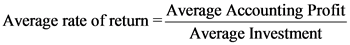# A company’s required rate of return. typically its cost of capital is called the:

A the company’s required rate of return, typically its cost of
capital is called the: Internal rate of return. Average rate of return. Hurdle rate. Maximum rate. Payback rate.

General guidance

Concepts and reason
Cost of capital: The cost of capital is one of the opportunity cost for a particular investment. It is rate of return that may be earned by investing the same amount into a different investment with the same risk. The cost of capital is the required rate of return that induces the investor to make an investment. Cost of capital is used as discounting rate in order to determine present value of estimated future cash flows.
Rate of return: The rate of return is the amount received from an investment over a particular period of time after deducting cost incurred on investment. The rate of return is the earnings that an asset generates in excess of its initial cost.

Fundamentals

Internal rate of return: Internal rate of return is the technique used in capital budgeting to measure the profitability and feasibility of investments. The discount rate at where the net present value becomes zero that rate is considered as IRR rate.
Payback rate: The rate at which the period that is recovered with the cost of investment is called as payback period.

Average rate of return: It is a rate of return that is used to measure the profitability of the investment. It does not take time value of one factor into consideration.

Step-by-step

Step 1 of 2

The internal rate of return is one of the investment decision techniques. The cost of capital is something that is not connected with the investment decision technique. The average rate of return is also profitability measure of investment one of the capital investment evaluation technique. Therefore, it also does not take the cost of capital into consideration. The payback rate is also measure of investment decision. Therefore, the above mentioned options are incorrect options.

All the options given internal rate of return, average rate of return and payback rate are profitability measure techniques, all these options do not relate to the cost of capital and cannot be termed as cost of capital.

Based on the given information in the question, identify the correct option that is called as cost of capital.

Step 2 of 2

Hurdle rate is the minimum required rate of return expected by the fund providers or investors of the company. This rate is used to compare with internal rate of return of the company and if IRR is greater than hurdle rate, the project is accepted. Therefore, the cost of capital is called as the hurdle rate.

The cost of capital is called as the hurdle rate.

The hurdle rate is the cost of capital which is compared with internal rate of return. If the hurdle rate is greater than the IRR rate, then the project is rejected. The hurdle rate is used as a comparison between the IRR rate and the required rate of return.